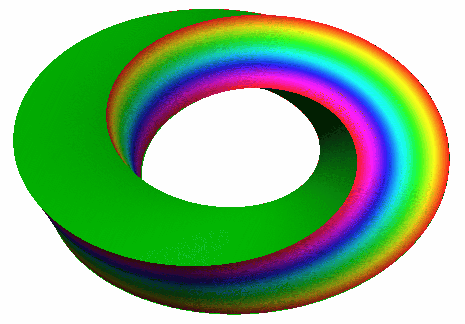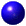Fine properties of stochastic processesCollaborative Research Centre 701
30 November 2009

Department of Mathematics , V3-201 (Common Room)

University of Bielefeld

Organizers
Moritz Kassmann and Alexander Teplyaev

The workshop is part of the conference program of the DFG-funded CRC 701 "Spectral Structures and Topological Methods in Mathematics" at the University of Bielefeld.
Invited speakersSteffen Dereich (Universität Marburg)Masha Gordina (University of Connecticut)Michael Hinz (Universität Jena)Alexander Lindner (TU Braunschweig)Martynas Manstavičius (Vilniaus Universitetas)Thomas Simon (Université de Lille)Toshihiro Uemura (Kansai University, Osaka)Jeannette Woerner (TU Dortmund)
*(to be confirmed)
Limited funds

Limited funds for support of PhD students/Postdocs (i.e. train tickets) are available.Steffen Dereich (Universität Marburg) Rough paths analysis of general Banach space-valued Wiener processes In the past the theory of rough paths has proven to be an elegant tool for deriving support theorems and large deviation principles. In this talk I will explain how this approach can be used in the analysis of stochastic flows generated by Kunita SDE's. As driving processes I will consider general Banach space valued Wiener processes.Masha Gordina (University of Connecticut) Levy processes in Lie groups This is a joint work with S.Albeverio. Classifying Levy processes on curved spaces is a difficult problem. The main obstacle is not having a natural Fourier transform. The choice of such depends on the structure of a Lie group. We use subordination to describe some Levy processes using different decompositions of the Lie groups.Michael Hinz (Universität Jena) Form Laplacians on Fractals Meanwhile an analysis on sufficiently simple fractal sets (such as Sierpinski gaskets or Koch curves) is well established and in many aspects well understood. In such cases one can construct Laplacians acting on functions on the fractals as the limits of rescaled sequences of discrete Laplacians on approximating graphs. In the case of a smooth Riemannian manifold of dimension \$n\$ on the other hand there exists a whole scale of \$k\$-Laplacians acting on differential \$k\$-forms, respectively, \$k=0,...,n\$. Already the \$1\$-Laplacian contains interesting and accessible geometric (curvature) information. Motivated by the search for curvature-like geometric quantities in the fractal case, we start an investigation of some analog of the \$1\$-Laplacian on some fractal sets. It involves simple tools from combinatorial geometry as well as from potential theory. The talk sketches some work in progress.Alexander Lindner (TU Braunschweig) On stationary distributions of some generalised Ornstein-Uhlenbeck processes. A generalised Ornstein-Uhlenbeck process is the solution V(t) to the stochastic differential equation V(t) = V(t-) dU(t) + dL(t), which is driven by the bivariate Levy process (U,L). We shall be interested in infinite divisibility and continuity properties of the marginal distribution of the stationary version of such processes. The case when U(t) is of the form (b-1) N(t) with a constant b in (0,1) and N and L are Poisson processes will be studied in detail, and it will be shown that continuity properties of the corresponding stationary distribution depends in an intrinsic way on algebraic properties of the constant b and on the ratio of the rates of the Poisson processes N and L. We shall also find new examples of b-decomposable distributions without their factor being infinitely divisible, or of non-infinitely divisible distributions with infinitely divisible symmetrisations. The talk is based on joint work with Ken-iti Sato.Martynas Manstavičius (Vilniaus Universitetas) On the p-variation of Linear Fractional Stable Motion Linear Fractional Stable Motion (LSFM) as defined in the monograph by G.~Samorodnicky and M.~Taqqu is one of interesting stochastic processes with trajectories having different global and local H\"older exponents. As a consequence of this, finding the p-variation index of such a process requires some additional work. In this talk, we will discuss a certain misconception regarding p-variation, a way of finding p-variation index of LFSM as well as some interesting and possibly difficult open questions regarding stable laws.Thomas Simon (Université de Lille) Absolute continuity of Ornstein-Uhlenbeck processes Let X be a multidimensional Ornstein-Uhlenbeck process, solution to the S.D.E. dX = AX + dB where A is a real nxn matrix and B a Lévy process. We show that when A is non-singular, the law of X_1 is absolutely continuous if and only if the jumping measure of B fulfils a certain geometric condition with respect to A and the Gaussian part of B, which we call the exhaustion property. This optimal criterion is much weaker than for B, which might be very singular and genuinely one-dimensional. The proof uses a certain time derivation procedure and basic arguments from controllability theory.Toshihiro Uemura (Kansai University, Osaka) Conservation property for symmetric jump processes I will consider the conservativeness of symmetric jump processes. Under a reasonable assumption on the jump kernel, the conservativess is shown. After proving the property for the 'trancated' jump process, the conservativeness of the 'whole' process is given by using a perturbation method. This is a joint work with Jun Masamune.Jeannette Woerner (TU Dortmund) Analyzing the fine structure of stochastic processes In the recent years starting from the Black-Scholes model in mathematical finance many different models either based on semimartingales, purely continuous, pure jump and a mixture of both, or fractional Brownian motion have been proposed in an attempt to capture the empirical facts of real data both in finance and physics, e.g.~heavy tails, skewness, excess kurtosis and long range dependence. We propose to include the fine structure into this analysis, which makes it possible to distinguish between semimartingales and fractional Brownian motion on the one hand and on the other hand determine the presence of jumps and measurement errors or market microstructure. We will compare two different approaches, one based on the regularity of the sample paths, the other one based on the correlation structure. For both methods we provide consistency and a distributional theory and apply them to high frequency financial data and turbulence data. Furthermore, the distributional theory for fractional Brownian motion based models provides some new insight in the scaling laws for turbulence data.